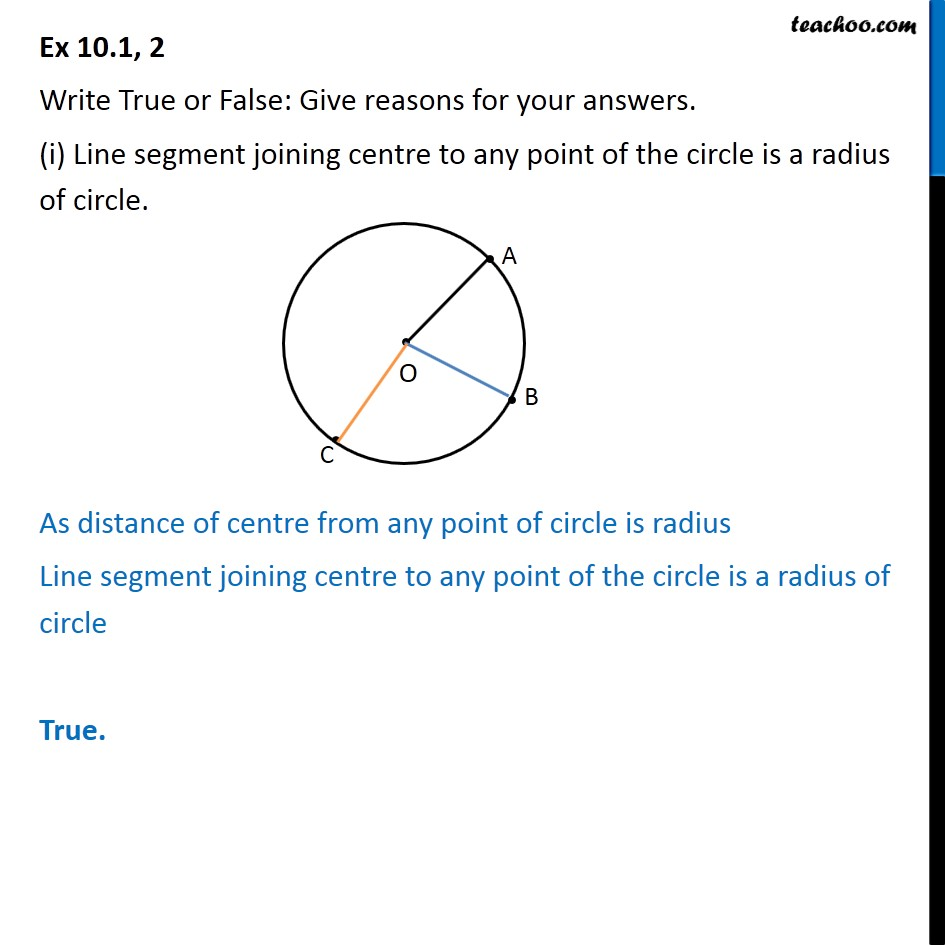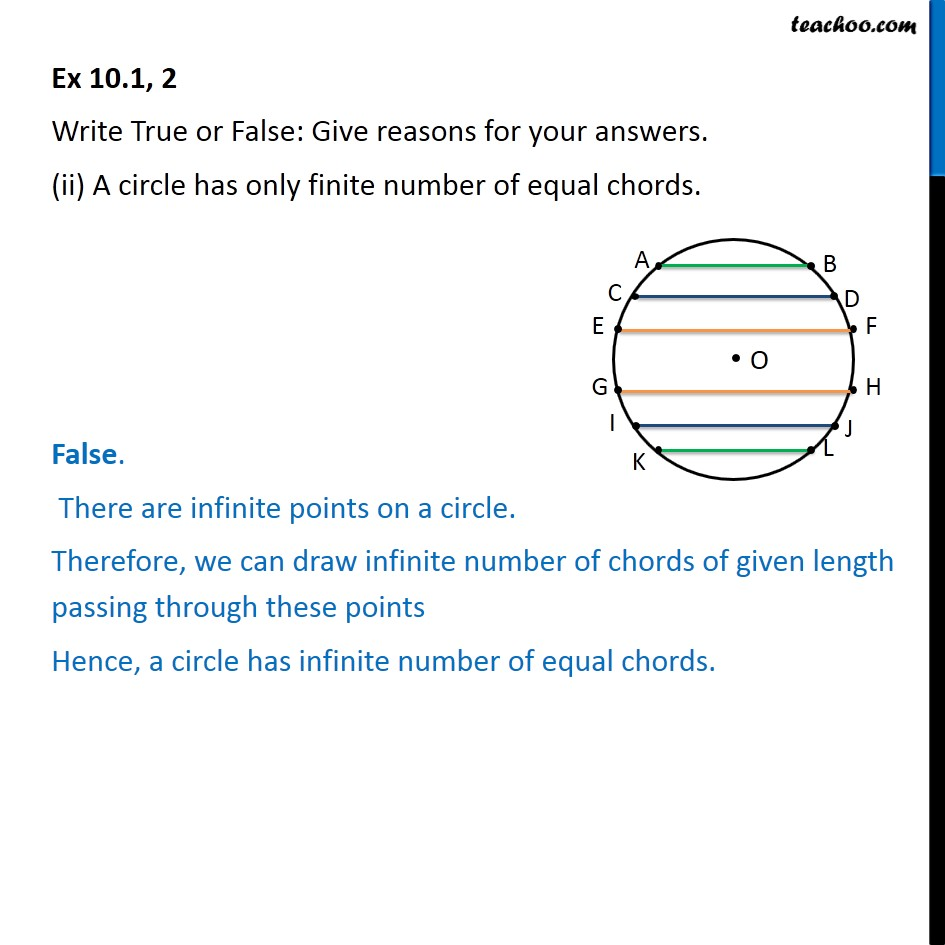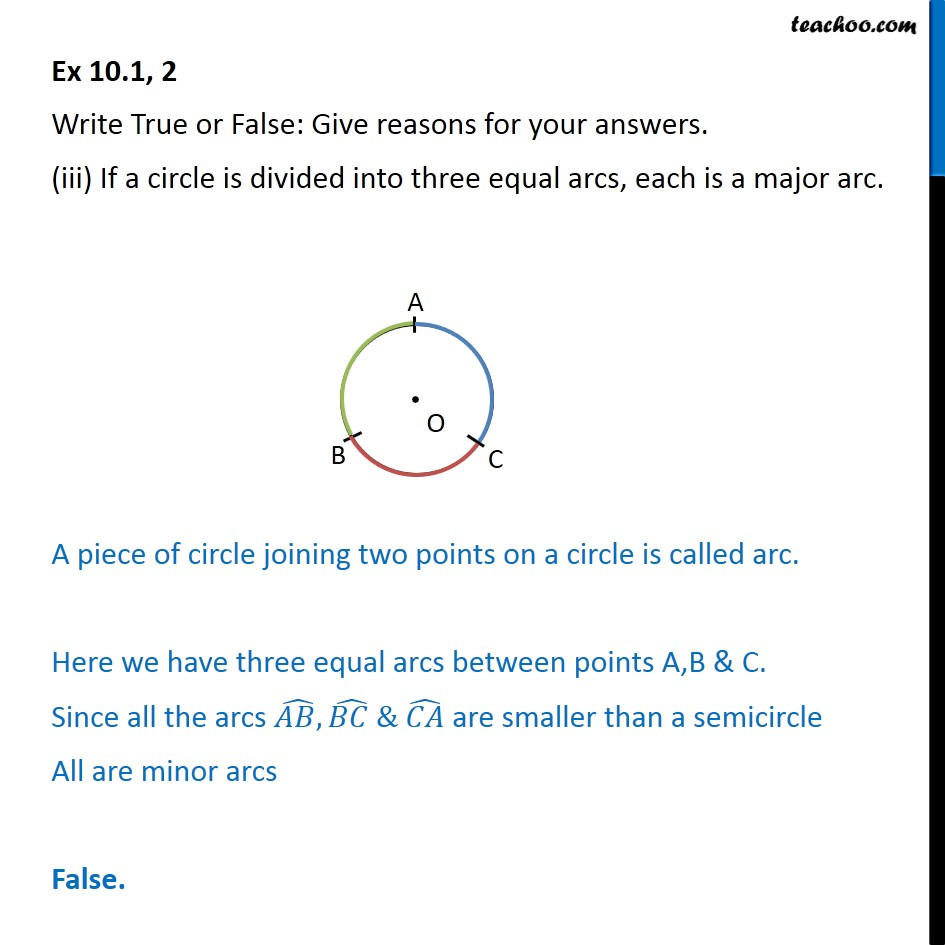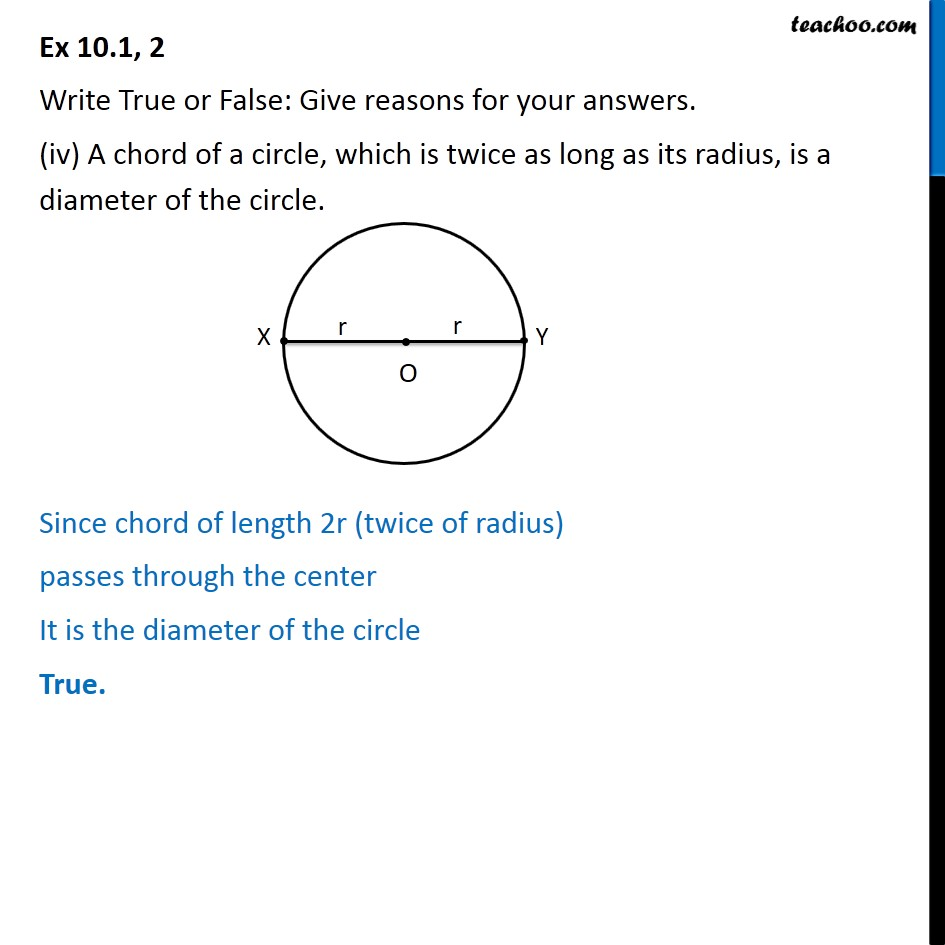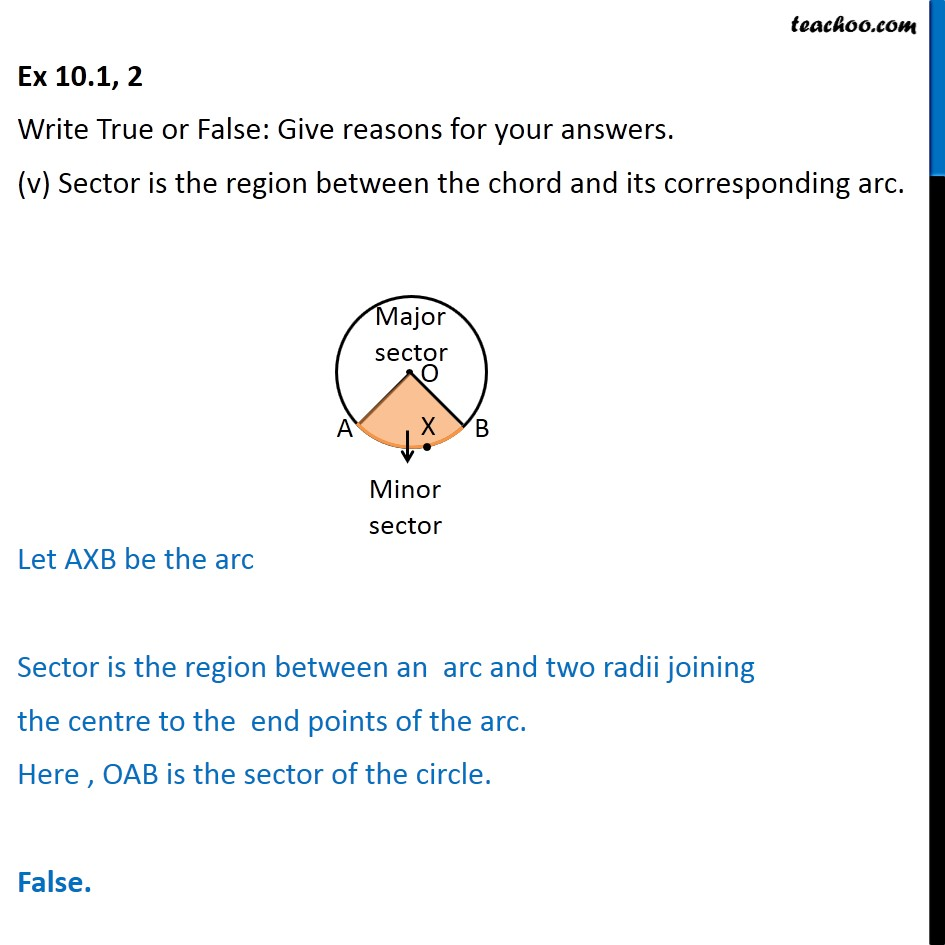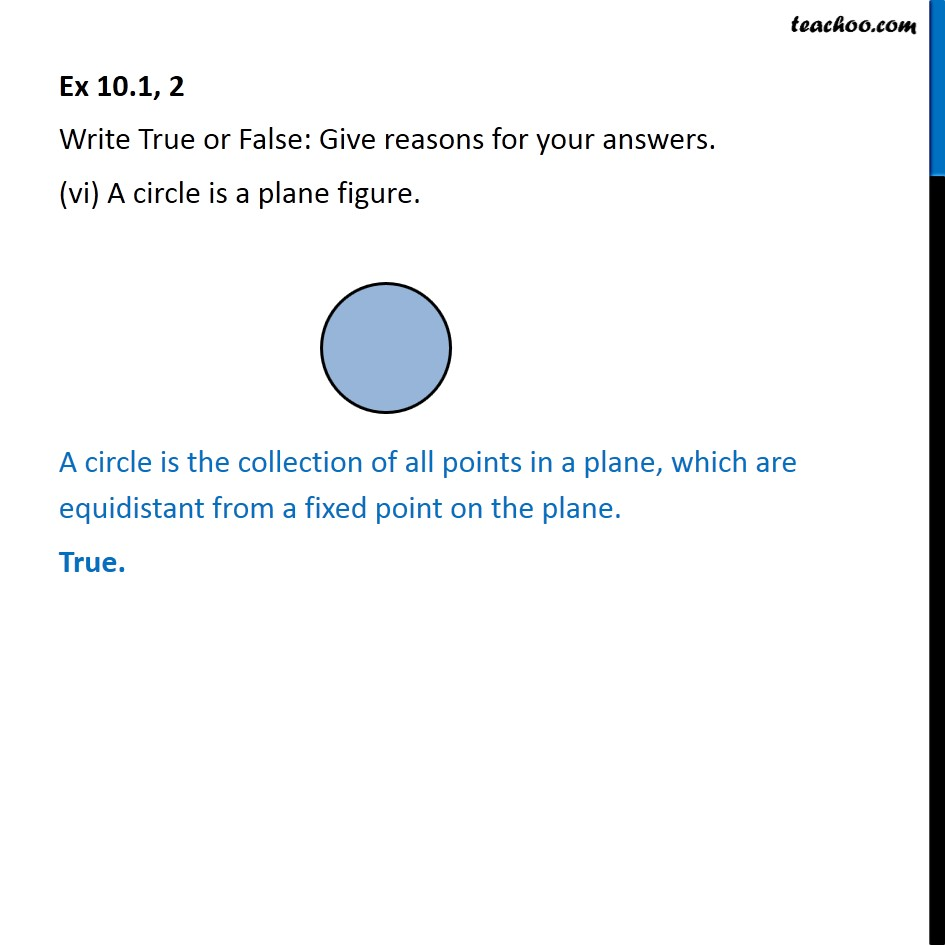1. Chapter 10 Class 9 Circles
2. Serial order wise
3. Ex 10.1

Transcript

Ex 10.1, 2 Write True or False: Give reasons for your answers. (i) Line segment joining centre to any point of the circle is a radius of circle. As distance of centre from any point of circle is radius Line segment joining centre to any point of the circle is a radius of circle True. Ex 10.1, 2 Write True or False: Give reasons for your answers. (ii) A circle has only finite number of equal chords. False. There are infinite points on a circle. Therefore, we can draw infinite number of chords of given length passing through these points Hence, a circle has infinite number of equal chords. Ex 10.1, 2 Write True or False: Give reasons for your answers. (iii) If a circle is divided into three equal arcs, each is a major arc. A piece of circle joining two points on a circle is called arc. Here we have three equal arcs between points A,B & C. Since all the arcs ( ,) ( ) & ( ) are smaller than a semicircle All are minor arcs False. Ex 10.1, 2 Write True or False: Give reasons for your answers. (iv) A chord of a circle, which is twice as long as its radius, is a diameter of the circle. Since chord of length 2r (twice of radius) passes through the center It is the diameter of the circle True. Ex 10.1, 2 Write True or False: Give reasons for your answers. (v) Sector is the region between the chord and its corresponding arc. Let AXB be the arc Sector is the region between an arc and two radii joining the centre to the end points of the arc. Here , OAB is the sector of the circle. False. Ex 10.1, 2 Write True or False: Give reasons for your answers. (vi) A circle is a plane figure. A circle is the collection of all points in a plane, which are equidistant from a fixed point on the plane. True.

Ex 10.1

Chapter 10 Class 9 Circles
Serial order wise

About the AuthorDavneet Singh
Davneet Singh is a graduate from Indian Institute of Technology, Kanpur. He has been teaching from the past 9 years. He provides courses for Maths and Science at Teachoo.